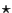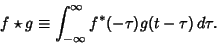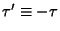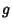## Cross-Correlation

Letdenote cross-correlation. Then the cross-correlation of two functionsandof a real variableis defined by(1)

wheredenotes Convolution andis the Complex Conjugate of. The Convolution is defined by(2)

therefore(3)

Let, soand(4)

The cross-correlation satisfies the identity(5)

Iforis Even, then(6)

wheredenotes Convolution.